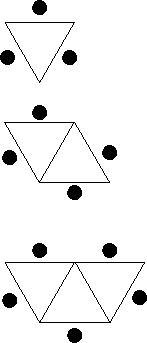# Triangular Tables

Alignments to Content Standards: 6.EE.B

A classroom has triangular tables. There is enough space at each side of a table to seat one child. The tables in the class are arranged in a row (as shown in the picture below).1. How many children can sit around 1 table? Around a row of two tables? Around a row of three tables?

2. Find an algebraic expression that describes the number of children that can sit around a row of $n$ tables. Explain in words how you found your expression.

3. If you could make a row of 125 tables, how many children would be able to sit around it?

4. If there are 26 children in the class, how many tables will the teacher need to seat all the children around a row of tables?

## IM Commentary

This task provides a good opportunity for group work and class discussions where students generate and compare equivalent expressions. In class discussion, students should be asked to connect the terms of an expression with quantities shown in the diagram. As part of the solution, students can make a table of values for increasing numbers of tables and seats and find a pattern. It is important that a discussion of the problem explicitly draws out how the diagram, the table of values, and the expressions are connected.

Part (c) of the question provides a good opportunity to discuss what might be a reasonable range of values for $n$, preparing students for the idea of the domain of a function later on.

## Solutions

Solution: Solution 1

1. Since one table has three sides and each seats one child, it follows that 3 children can sit around 1 table.

When two tables are put together in a row as pictured, then we can count the number of open sides around the perimeter of the two tables together, since an open side means one child can sit there. There are 4 sides that are open around the table, and so 4 children can sit around a row of 2 tables.

Using the same method as above, we see that when 3 tables are put into a row we will have 5 open sides around the tables. So, 5 children can sit around a row of 3 tables.2. To find an expression that describes the number of children that can sit around a row of $n$ tables, we can consider the diagram below. We see that we can fit 1 child at each horizontal table side (black dots) plus 1 child on the left and one on the right (white dots). So we have:

$$\text{children that can sit at } n \text{ tables} = n + 1 + 1 = n + 2$$Another way to think about counting seats is shown in the picture below. The first table seats two children and the last table seats two children (white dots). All other $n - 2$ tables seat one child (black dots). So we have:

$$\text{children that can sit at } n \text{ tables} = 2 \cdot 2 + (n - 2) \cdot 1 = 4 + (n - 2).$$Other expressions are also possible, which are all equivalent to $n + 2$.

3. Using our expression from part (b), with $n=125$, we see that

$$n + 2 = 125 + 2 = 127,$$

so 127 children can sit around a row of 125 tables.

4. Using our expression from before we know that a row of $n$ tables seats $n + 2$ children. If we want to seat 26 children we need to find n such that $n + 2 = 26$. So we have $n = 24$, which means that the teacher needs 24 tables to seat all students in the class.

Solution: Solution 2

1. See Solution 1
2. We can summarize the numbers we found in the solution above in a table of values:

number of tables, $n$ number of seats
1 3
2 4
3 5

Looking at a few more examples, with 4 and 5 tables forming a row, we can extend our table of values:

number of tables, $n$ number of seats
1 3
2 4
3 5
4 6
5 7

We notice the pattern, that the number of seats is equal to the number of tables plus 2. So we have:

$$\text{children that can sit at } n \text{ tables} = n + 2.$$
3. See Solution 1
4. See Solution 1